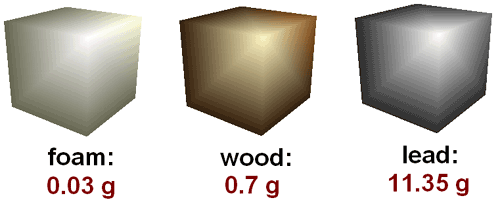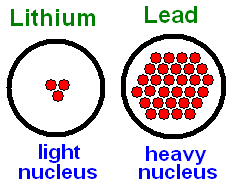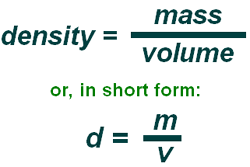## Microsoft word - density - english.docA Definition Of Density
vrij naar: http://www.green-planet-solar-energy.com/definition-of-density.html
It is useful to have a clear definition of density, as this is perhaps not easy to understand. We are all familiar with weight, and we are all familiar with volume, which is the amount of space that something takes up. We work with these ideas every day of our lives, yet we almost never reflect on the density of objects. A Definition Of Density
In short, density is how much something of a given volume weighs. This is
generally measured by taking a cubic centimeter of a substance. If we take a
cubic centimeter of some commonly known substances, we can see that they
So from the above examples it is easy to see that some of the cubes weigh more than others, even though they all take up the same amount of space. This agrees with our experience. We know that a huge block of foam is easier to lift than the same size block of wood or metal. Now we can see that the foam
block is less dense than the others, making it easier to lift. We can all imagine
lifting a cube of foam, but try lifting the same cube of lead or steel!
Calculating Density
The best way to calculate the density of any material is to take a cubic centimeter of it and weigh it. That will give you the mass of the substance per
cubic centimeter
. For lead (Pb), each cubic centimeter of it weighs 11.35
grams, making its density 11.35 grams per cubic centimeter, or g/cm^3 if you're
scientifically minded.
For lead, 1 cubic centimeter weighs 11.35 grams, while 2 grams weighs 22.70 grams and so on; so we see that the ratio of 11.35 grams per cubic centimeter is constant.The Formula For Density
"Per" is the maths word for divided by. So if we translate the units of density, which are grams per cubic centimeter, into math speak, we get grams / cubic centimeter, or g / cm^3, also written as gcm^-3. density by
dividing mass by volume as shown to
the right. The units can actually be of any
kind, as long as they are the same
between substances which have to be
compared. We could measure density in
milligrams per cubic mile, or kilotons per cubic nanometer, it makes no difference. As long as the units are clearly stated, conversion to standard units is easy. Why are some things denser than others?
This is the big question. A material that is denser has more matter, or stuff,
packed into the same space. To understand this fully, we need to consider matter
in terms of the atoms that it is made of. The more stuff that's in the nuclei of the
atoms of the substance, the greater the density will be.
See how this works in the very simplified picture of two atoms below. The mass of the substances is held in the nuclei, and even though the metals take up the same amount of space, the one on the left (Lithium) has far less
matter in its nucleus than the lead on the right. It is because of this, and this
alone, that lead is more dense than Lithium metal.